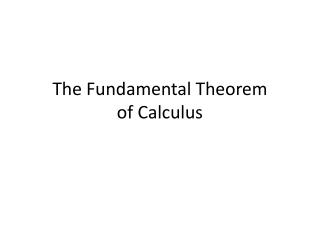# The Fundamental Theorem of Calculus - PowerPoint PPT PresentationDownload PresentationThe Fundamental Theorem of Calculus

The Fundamental Theorem of CalculusDownload Presentation## The Fundamental Theorem of Calculus

- - - - - - - - - - - - - - - - - - - - - - - - - - - E N D - - - - - - - - - - - - - - - - - - - - - - - - - - -
##### Presentation Transcript

1. The Fundamental Theorem of Calculus

2. Isaac Newton1642 - 1727 • 1702 portrait by Kneller • The original is in the National Portrait Gallery in London

3. On 16 July 1730 in Mathematics Alexander Pope (1688-1744) published the famous lines which were intended as an epitaph for Newton: Nature and Nature's Laws lay hid in night: God said, Let Newton be!, and all was light. They were published in the Grub-Street Journal.

4. Took Descartes’s Geometry in hand, tho he had been told it would be very difficult, read some ten pages in it, then stopt, began again, went a little farther than the first time, stopt again, went back again to the beginning, read on til by degrees he made himself master of the whole, to that degree that he understood Descartes’s Geometry better than he had done Euclid.

5. Descartes’s Geometry, 1637, 1659

6. van Heurat on Arc Length, 1661

7. van Heuraet’s rectification, 1659

8. The Fundamental Theorem of Calculus • A Method whereby to square such crooked lines as may be squared.

9. For Newton • Mathematical quantities are described by Continuous Motion. • E.g., Curves are generated by moving points • In Modern Terms: All variables are functions of time

10. Newton said that quantities flow, and so called them fluents. • How fast they flow – or flex – he called fluxions. • Par abuse de langu, d/dt ( fluent ) = fluxion

11. The Product Rule: 11 November 1675 • Leibniz introduces the dx notation and asks “Is dx . dy = d(x . y)?” • He lets x = cz + d and y = z2 + bz and computes dx.dy = c (2z +b). • “But you get the same thing if you work out d(x.y) in a straightforward manner.” • Later that day: Whoops!

12. The Product Rule: 21 November 1675 • dx . y = d (x . y) – x . dy. • “Now this is a really noteworthy theorem.”* • It took Leibniz 10 days to correct his error, and, my dear students, if you don’t master this in 10 days, you will not do well on the next exam. • From the zeroeth Putnam Exam (1933): When is dx . dy = d(x . y)?” * The Early Mathematical Manuscripts of Leibniz, ed. by J. M. Child (1920/2005), p. 107

13. Bonaparte rightly said that many of the decisions faced by the commander-in-chief resemble mathematical problems worthy of the gifts of a Newton or an Euler. • Carl von Clausewitz, On War, 1832